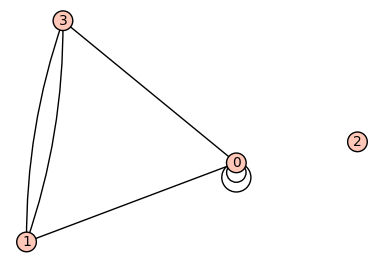# Walks in graphs¶

This section provides some examples on Chapter 1 of Stanley’s book [Stanley2013].

We begin by creating a graph with 4 vertices:

sage: G = Graph(4)
sage: G
Graph on 4 vertices


This graph has no edges yet:

sage: G.vertices()
[0, 1, 2, 3]
sage: G.edges()
[]


Before we can add edges, we need to tell Sage that our graph can have loops and multiple edges.:

sage: G.allow_loops(True)
sage: G.allow_multiple_edges(True)


Now we are ready to add our edges by specifying a tuple of vertices that are connected by an edge. If there are multiple edges, we need to add the tuple with multiplicity.:

sage: G.add_edges([(0,0),(0,0),(0,1),(0,3),(1,3),(1,3)])


Now let us look at the graph!

sage: G.plot()
Graphics object consisting of 11 graphics primitivesWe can construct the adjacency matrix:

sage: A = G.adjacency_matrix()
sage: A
[2 1 0 1]
[1 0 0 2]
[0 0 0 0]
[1 2 0 0]


The entry in row $$i$$ and column $$j$$ of the $$\ell$$-th power of $$A$$ gives us the number of paths of length $$\ell$$ from vertex $$i$$ to vertex $$j$$. Let us verify this:

sage: A**2
[6 4 0 4]
[4 5 0 1]
[0 0 0 0]
[4 1 0 5]


There are 4 paths of length 2 from vertex $$0$$ to vertex $$1$$: take either loop at $$0$$ and then the edge $$(0, 1)$$ (2 choices) or take the edge $$(0, 3)$$ and then either of the two edges $$(3, 1)$$ (two choices):

sage: (A**2)[0,1]
4


To count the number of closed walks, we can also look at the sum of the $$\ell$$-th powers of the eigenvalues. Even though the eigenvalues are not integers, we find that the sum of their squares is an integer:

sage: A.eigenvalues()
[0, -2, 0.5857864376269049?, 3.414213562373095?]
sage: sum(la**2 for la in A.eigenvalues())
16.00000000000000?


We can achieve the same by looking at the trace of the $$\ell$$-th power of the matrix:

sage: (A**2).trace()
16